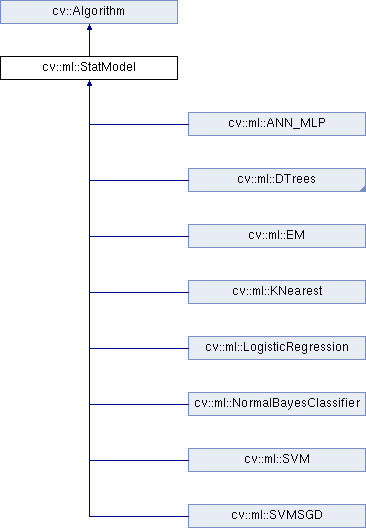OpenCV  3.2.0 Open Source Computer Vision
cv::ml::StatModel Class Referenceabstract

Base class for statistical models in OpenCV ML. More...

#include "ml.hpp"

Inheritance diagram for cv::ml::StatModel:## Public Types

enum  Flags {
UPDATE_MODEL = 1,
RAW_OUTPUT =1,
COMPRESSED_INPUT =2,
PREPROCESSED_INPUT =4
}

## Public Member Functions

virtual float calcError (const Ptr< TrainData > &data, bool test, OutputArray resp) const
Computes error on the training or test dataset. More...

virtual bool empty () const
Returns true if the Algorithm is empty (e.g. in the very beginning or after unsuccessful read. More...

virtual int getVarCount () const =0
Returns the number of variables in training samples. More...

virtual bool isClassifier () const =0
Returns true if the model is classifier. More...

virtual bool isTrained () const =0
Returns true if the model is trained. More...

virtual float predict (InputArray samples, OutputArray results=noArray(), int flags=0) const =0
Predicts response(s) for the provided sample(s) More...

virtual bool train (const Ptr< TrainData > &trainData, int flags=0)
Trains the statistical model. More...

virtual bool train (InputArray samples, int layout, InputArray responses)
Trains the statistical model. More...Public Member Functions inherited from cv::Algorithm
Algorithm ()

virtual ~Algorithm ()

virtual void clear ()
Clears the algorithm state. More...

virtual String getDefaultName () const

virtual void read (const FileNode &fn)
Reads algorithm parameters from a file storage. More...

virtual void save (const String &filename) const

virtual void write (FileStorage &fs) const
Stores algorithm parameters in a file storage. More...

## Static Public Member Functions

template<typename _Tp >
static Ptr< _Tp > train (const Ptr< TrainData > &data, int flags=0)
Create and train model with default parameters. More...Static Public Member Functions inherited from cv::Algorithm
template<typename _Tp >
static Ptr< _Tp > load (const String &filename, const String &objname=String())
Loads algorithm from the file. More...

template<typename _Tp >
static Ptr< _Tp > loadFromString (const String &strModel, const String &objname=String())
Loads algorithm from a String. More...

template<typename _Tp >
static Ptr< _Tp > read (const FileNode &fn)
Reads algorithm from the file node. More...Protected Member Functions inherited from cv::Algorithm
void writeFormat (FileStorage &fs) const

## Detailed Description

Base class for statistical models in OpenCV ML.

## § Flags

Predict options

Enumerator
UPDATE_MODEL
RAW_OUTPUT

makes the method return the raw results (the sum), not the class label

COMPRESSED_INPUT
PREPROCESSED_INPUT

## § calcError()

 virtual float cv::ml::StatModel::calcError ( const Ptr< TrainData > & data, bool test, OutputArray resp ) const
virtual

Computes error on the training or test dataset.

Parameters
 data the training data test if true, the error is computed over the test subset of the data, otherwise it's computed over the training subset of the data. Please note that if you loaded a completely different dataset to evaluate already trained classifier, you will probably want not to set the test subset at all with TrainData::setTrainTestSplitRatio and specify test=false, so that the error is computed for the whole new set. Yes, this sounds a bit confusing. resp the optional output responses.

The method uses StatModel::predict to compute the error. For regression models the error is computed as RMS, for classifiers - as a percent of missclassified samples (0%-100%).

## § empty()

 virtual bool cv::ml::StatModel::empty ( ) const
virtual

Returns true if the Algorithm is empty (e.g. in the very beginning or after unsuccessful read.

Reimplemented from cv::Algorithm.

## § getVarCount()

 virtual int cv::ml::StatModel::getVarCount ( ) const
pure virtual

Returns the number of variables in training samples.

## § isClassifier()

 virtual bool cv::ml::StatModel::isClassifier ( ) const
pure virtual

Returns true if the model is classifier.

## § isTrained()

 virtual bool cv::ml::StatModel::isTrained ( ) const
pure virtual

Returns true if the model is trained.

## § predict()

 virtual float cv::ml::StatModel::predict ( InputArray samples, OutputArray results = noArray(), int flags = 0 ) const
pure virtual

Predicts response(s) for the provided sample(s)

Parameters
 samples The input samples, floating-point matrix results The optional output matrix of results. flags The optional flags, model-dependent. See cv::ml::StatModel::Flags.

Implemented in cv::ml::LogisticRegression.

## § train() [1/3]

 virtual bool cv::ml::StatModel::train ( const Ptr< TrainData > & trainData, int flags = 0 )
virtual

Trains the statistical model.

Parameters
 trainData training data that can be loaded from file using TrainData::loadFromCSV or created with TrainData::create. flags optional flags, depending on the model. Some of the models can be updated with the new training samples, not completely overwritten (such as NormalBayesClassifier or ANN_MLP).

## § train() [2/3]

 virtual bool cv::ml::StatModel::train ( InputArray samples, int layout, InputArray responses )
virtual

Trains the statistical model.

Parameters
 samples training samples layout See ml::SampleTypes. responses vector of responses associated with the training samples.

## § train() [3/3]

template<typename _Tp >
 static Ptr<_Tp> cv::ml::StatModel::train ( const Ptr< TrainData > & data, int flags = 0 )
static

Create and train model with default parameters.

The class must implement static create() method with no parameters or with all default parameter values

The documentation for this class was generated from the following file: